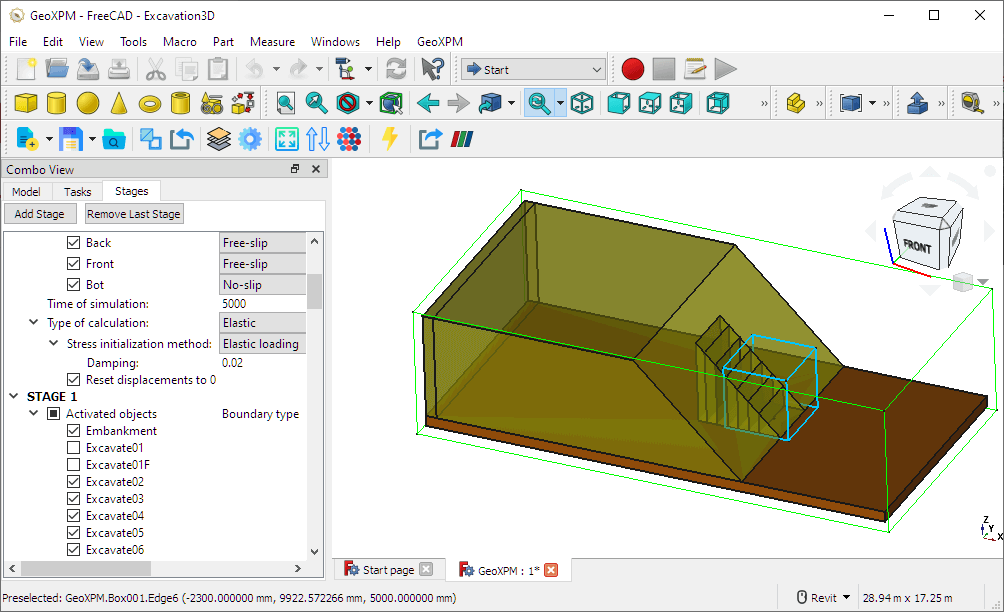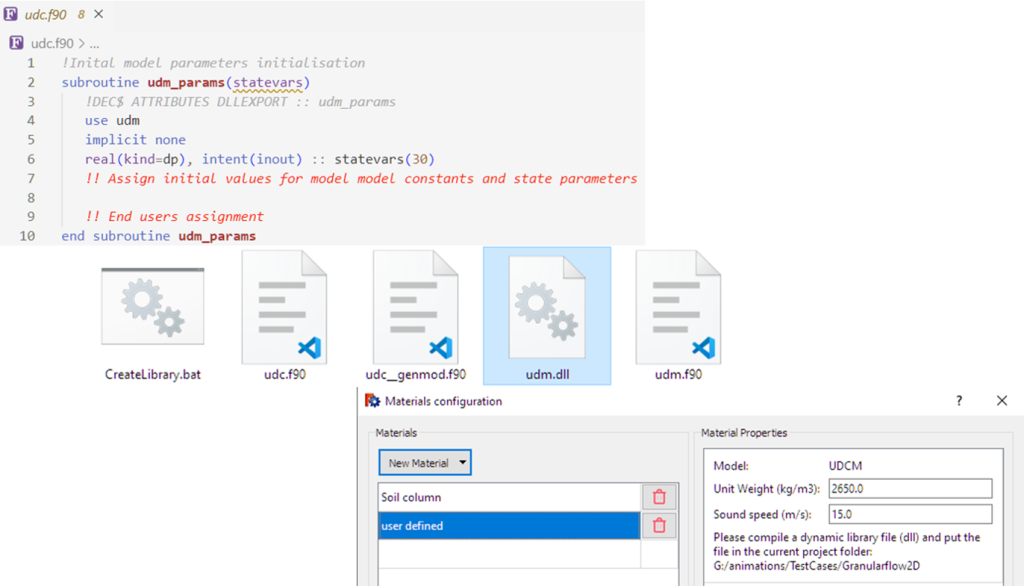# Solving extreme geotechnical problems involving large deformations

wikipedia.org

New tutorials are coming frequently!

#### General User Interface Explained

In this tutorial. We will explain about:

• GeoXPM interface, including Toolbox, Menu, and Tabs
• The general workflow to solve a geotechnical problem.

GeoXPM_UIUX pdf#### 2D Granular Flow

In this tutorial. We are going to simulate a simple 2D granular flow problem. You will learn how to:

• Draw primitive geometry.
• Define SPH parameters and materials.
• Define particle generate order.
• Define construction stages.
• Run simulation and visualise data.

2D Granular flow tutorial pdf

Sample 2D Granular Flow project files

#### 3D Granular Flow

In this tutorial. We are going to simulate a simple 3D granular flow problem. You will learn about the differences between working in 2D (previous example) and 3D in GeoXPM.

3D Granular flow tutorial pdf

Sample 3D Granular Flow project files

#### 3D Retrogressive Failure

In this tutorial. We are going to demonstrate the strength of GeoXPM through a 3D retrogressive failure of sensitive clays (or quick clays). You will learn about behaviours of soils using different constitutive models.

3D Retrogressive failure of sensitive Clay tutorial pdf

#### 3D Hourglass Simulation

In this tutorial, we are going to create a simulation of granular flow in an hourglass. You will learn about:

• Working with mesh objects.
• Creating a basic rendering with path tracing.

3D Hourglass tutorial pdf

Sample 3D Hourglass project files

#### 3D Staged Excavation

In this tutorial. We are going to model an excavation process where the soil slope is slowly excavated until collapse. Through this example, you will learn about:

• Drawing and manipulating 3D objects.
• Setting up construction stages.
• Data post-processing.

3D Excavation tutorial pdf

Sample 3D Excavation project files

#### 3D Terrain Debris Flow

In this tutorial. We are going to conduct a large scale simulation of debris flow problem. The initial geometry will be imported from a digital elevation model. You will learn about:

• Working with real-world data.
• Resources to obtain terrain from all over the world.
• Creating animation sequences.

3D Terrain tutorial pdf

Sample 3D Terrain project files

#### User-defined Constitutive Model

In this tutorial, we will explore the process of creating a custom constitutive model (material model) and incorporating it into GeoXPM for advanced simulations. It is important to note that this is an advanced topic that requires a certain level of familiarity with coding in Fortran.

• Throughout the tutorial, you will gain knowledge on Fortran templates, compiling dynamic linked libraries, and integrating your user-defined model into the GeoXPM interface.

GeoXPM User-defined Constitutive Model pdf

GeoXPM User-defined Constitutive Model Template